## Calculating Weighted Average in Excel

Weighted average is very useful in data analysis and reporting. It paints a clearer picture of your dataset and will transform data into more accurate information. In this article, we will go through how weighted average can be calculated in Excel and go through some examples. But first we will go through what weighted average is and when we should use it.

## What is Weighted Average?

Weighted average is the calculation of averages except we can take into account the importance of each value in the dataset. When we learn how to calculate averages at school, there is an assumption that each number in the dataset has equal weighting. However this is very often not how the real world works.

For example, let’s say we want to calculate and analyze the results of a survey we sent out and the survey has 10 questions, if we add the results up and divide by 10, we would be assuming that all 10 questions are of equal importance. In actual fact, the results and responses we get from question one and two could be twice or four times as important as the other questions. By calculating weighted averages, we can get much more meaningful information and a more accurate picture of what the data is showing us.

## How To Calculate Weighted Averages in Excel?

To clearly illustrate the impact weightings have on weighted averages, we will go through one example where the values have equal weightings and another example where the values have very extreme opposite weightings (e.g. 10% and 90%). The formulas are exactly the same but we will go through both scenarios 1) where weights are presented as percentages and 2) where weightings are presented simply as numbers. This is how it is calculated:

• Multiply each value by its weighting
• Divide by total by the sum of the weightings
• Note: if weightings are presented as percentages, the sum of the weightings should add up to 100%. That means we will just be dividing the total by 1. So it’s optional whether we perform this last step. It is probably more important that we check the percentages do add up to 100%.

### SUMPRODUCT Function

Before we continue, we will briefly explain how the SUMPRODUCT function works in Excel because it just makes the calculation of weighted averages so much easier. As mentioned above, to calculate weighted averages, we will need to multiply each value by its weighting and then add up the results. You can probably guess from the name of the function: SUMPRODUCT, this function can do exactly that.

This function is particularly useful when we have large arrays of data:

The way SUMPRODUCT function works is that users can enter multiple arrays into the formula:

=SUMPRODUCT(array 1, [array 2], [array 3]…)

Notice that array 2 and 3 and so on are in square brackets. This means they are optional fields. In cases where only one array is entered, the SUMPRODUCT function will simply add up the numbers in that array and return the result.

In cases where there are multiple arrays, the image below would best explain how the function works: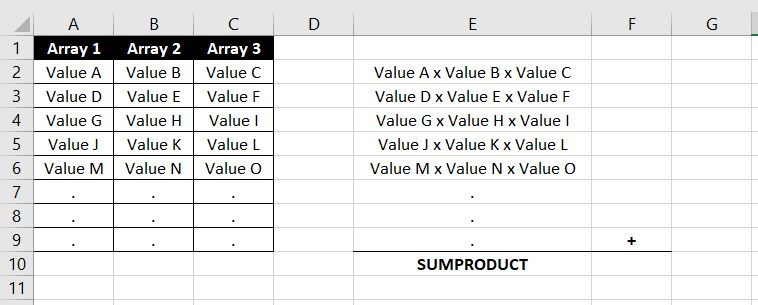The first value of each array will be multiplied together and the second of each array and so on… At the end all the results will be added together and this is the value the SUMPRODUCT function will return.

Note that it is important each array contains the same number of values. To illustrate the issue, let’s have a look at below: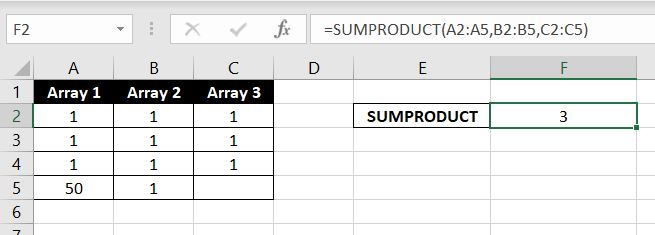The SUMPRODUCT returned a value of 3. The first values of each array is 1 so 1 x 1 x 1 = 1. This is the same for 2nd and 3rd row for each array. However there are only 3 numbers in array 3 which means the 4th value is 0. Hence Excel performs its usual calculation 50 x 1 x 0 = 0. And at the end the SUMPRODUCT function adds up all the results (1 + 1 + 1 + 0) and returns 3.

There is also the possibility of getting #VALUE for SUMPRODUCT. This is when the number of values for each array does not match: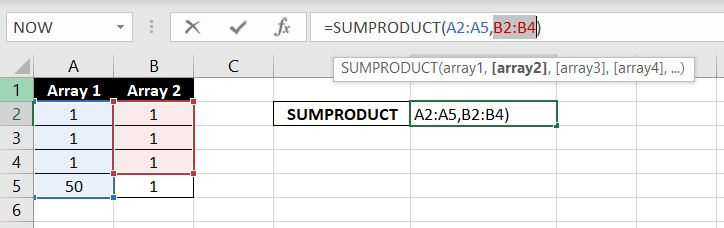Because array 1 has 4 values and array 2 only has 3 values, there is no corresponding number in array 2 for the number in array 1 to multiply with. As a result, SUMPRODUCT returns #VALUE.

### Weighted Average Based On Percentages

To illustrate the difference, let’s first go through an example where the values are of equal importance: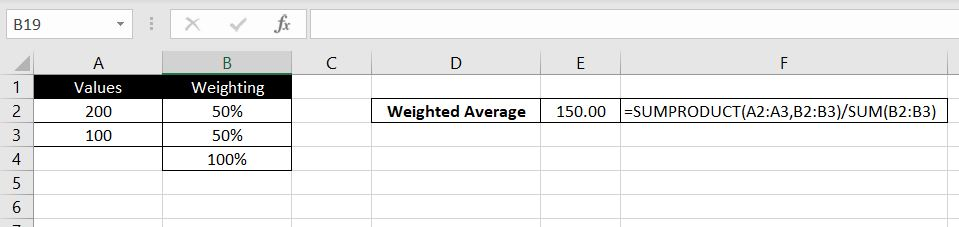In this case both numbers are of equal weightings. This means we could have simply performed the calculation as if it was a standard arithmetic average:

=(200 + 100)/2

And this of course would also equal 150.

Now let’s consider this example where the weighting for the first value is 9x that of the other value: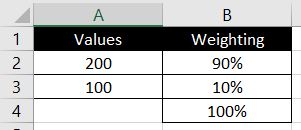Applying the formula – first we use SUMPRODUCT function to multiply each value by its respective weighting and add up the result. Then we divide this result by the sum of the weightings (90% + 10%)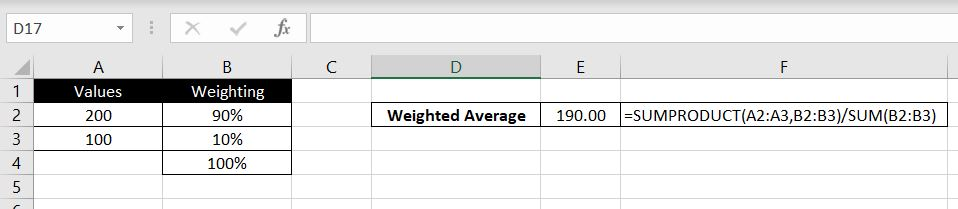Because the weighting for the first value is 90% while the weighting for the second value is only 10%, we can see how the weighted average is heavily skewed towards the first value. Now let’s have a look at how we can calculate weighted averages when weightings are not presented as percentages but as numbers.

### Weighted Average Based on Weightings

The formula is exactly the same. In this case we will put a much higher weighting on the second value: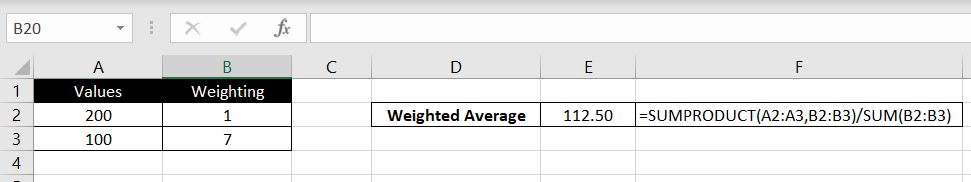And as a result, the weighted average is now much more heavily skewed towards the second value instead of the first.

We hope you now understand why weighted averages are useful and how they can be calculated. If you need any further explanation, please feel free to leave a comment below.